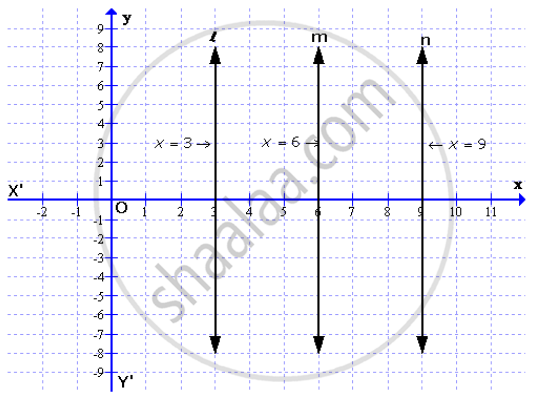# On a Graph Paper, Draw the Line X = 6. Now, on the Same Graph Paper, Draw the Locus of the Point Which Moves in Such a Way that Its Distantce from the Given Line is Always Equal to 3 Units - Mathematics

Sum

On a graph paper, draw the line x = 6. Now, on the same graph paper, draw the locus of the point which moves in such a way that its distantce from the given line is always equal to 3 units

#### SolutionOn the graph, draw axis XOX’ and YOY’
Draw a line l, x = 6 which is parallel to y-axis
Take points P and Q which are at a distance of 3 units from the line l.
Draw lines m and n from P and Q parallel to l
With locus = 3, two lines can be drawn x = 3 and x = 9.

Concept: Constructions Under Loci
Is there an error in this question or solution?

#### APPEARS IN

Selina Concise Maths Class 10 ICSE
Chapter 16 Loci (Locus and Its Constructions)
Exercise 16 (A) | Q 20 | Page 238